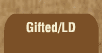Mathematics and Dyslexia

Tips for Learning the Times Tables

Tools for the Times Tables & Workbook

Home > Math > times tables practice > Sevens (big, in order)

## The Seven Times Tables In Order - second set

You should know7 x 6, 7 x 9 and 7 x 10 already.

Not too many more to learn --

1. 7 x 6=
2. 7 x 7=
3. 7 x 8=
4. 7 x 9=
5. 7 x 10=
6. 10 x 7=
7. 9 x 7=
8. 8 x 7=
9. 7 x 7=
10. 6 x 7=
11. 7 x 7=
12. 7 x 8=
13. 9 x 7=
14. 7 x 6=
15. 8 x 7=